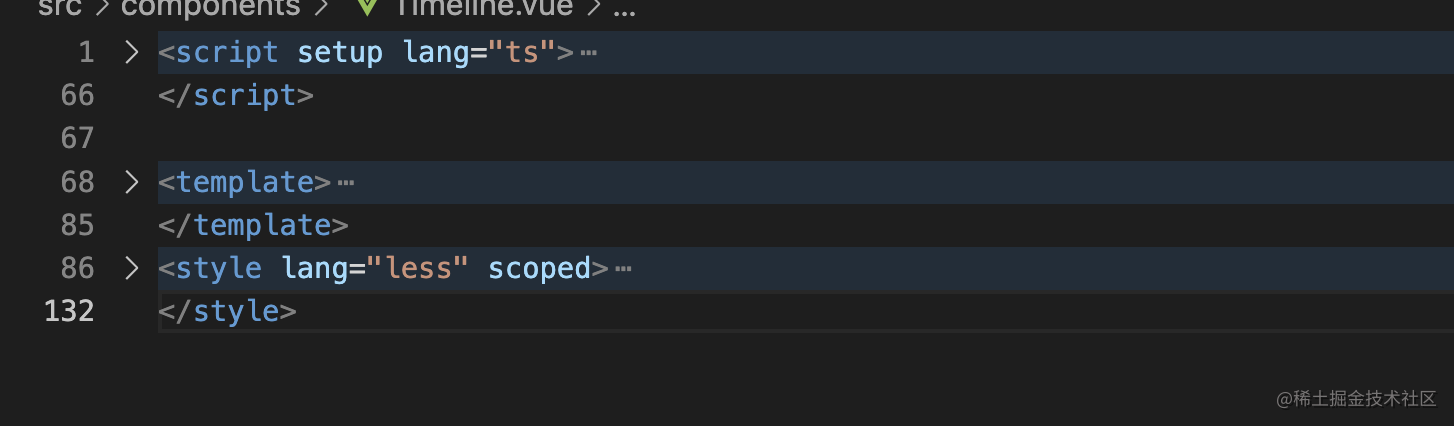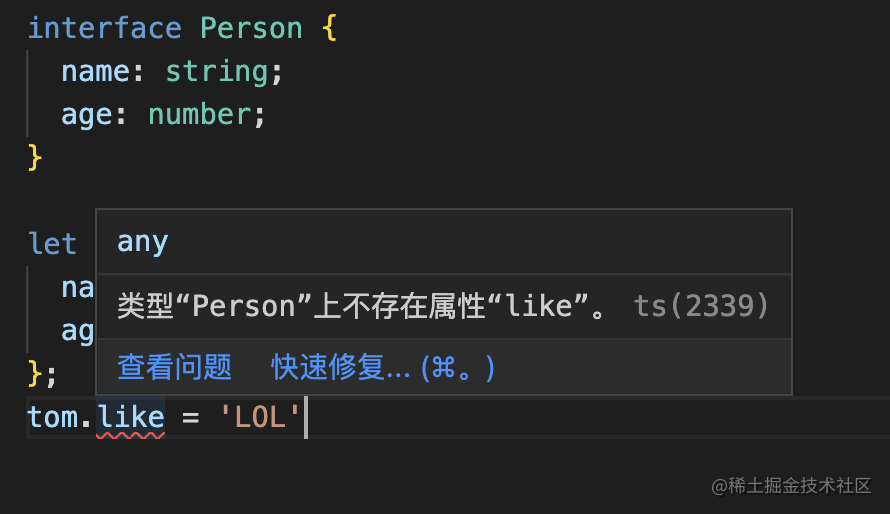# 项目中用到的TS## ts环境搭建（基础环境/vue3环境）

#### 1、ts编译

npm install -g `typescript`

`tsc xxx.ts`

#### 2、vue中使用

vue3中正常安装即包含了ts（vue3本身就使用了ts编译）。## 常用的原始类型、类型推断、联合类型

``````TS官网：原始数据类型包括：布尔值、数值、字符串、`null`、`undefined` 以及 ES6 中的新类型 [`Symbol`]和 ES10 中的新类型 [`BigInt`]

``````let bol = false;
let num = 0;
let str = '';

let bol:boolean = false;
let num:number = 0;
let str:string = '';

`联合类型`：在声明变量时候，使用 `|` 分隔每个类型。

``````let myFavoriteNumber: string | number;
myFavoriteNumber = 'seven';
myFavoriteNumber = 7;

## 接口是什么以及接口的使用方法

#### 1、接口是什么

``````interface Person {
name: string;
age: number;
}

let tom: Person = {
name: 'Tom',
age: 25
};

tom.like = 'LOL'当你向tom添加新属性时候，会提示类型Person上不存在新属性，或者修改现有属性但是类型不会。

#### 2、接口常用来做什么？

`接口`的用来做什么呢？接口解释时候说：接口用于`对象的形状`。所以可以从此入手。当我们得到了一个需求。

``````interface Person {
name: string;
eat: string;
like: string;
age: number;
}
let xiaoming:Person = {
name: '小明',
like: 'eat 胡建人',
eat: '胡建人',
age: 9527,

## 数组基础类型以及数组和接口的联合使用

#### 1、数组基础类型表示法【`类型+方括号`】

``````  let fibonacci: number[] = [1, 1, 2, 3, 5];
let fibonacci: string[] = ['str1','str2'];
let fibonacci: boolean[] = [true,false];

#### 2、数组基础类型表示法【`数组泛型`】，泛型可以看作是另外一种写法。

`````` let fibonacci Array<number> = [1, 1, 2, 3, 5];
let fibonacci Array<string> = ['str1','str2'];
let fibonacci Array<boolean> = [true,false];

#### 3、数组和接口的联合使用

``````interface Person {
name: string;
eat: string;
like: string;
age: number;
}

let person: Person[] = [
{
name: 'xiaoming',
eat: '福建人',
like: 'eat 福建人',
age: 9527,
}
]

let person: Array<Person> = [
{
name: 'xiaoming',
eat: '福建人',
like: 'eat 福建人',
age: 9527,
}
]

## 类型断言

``````interface Cat {
name: string;
run(): void;
}
interface Fish {
name: string;
swim(): void;
}

function isFish(animal: Cat | Fish) {
if (typeof animal.swim === 'function') {
return true;
}
return false;
}

// index.ts:11:23 - error TS2339: Property 'swim' does not exist on type 'Cat | Fish'.
//   Property 'swim' does not exist on type 'Cat'.

interface Cat {
name: string;
run(): void;
}
interface Fish {
name: string;
swim(): void;
}

function isFish(animal: Cat | Fish) {
if (typeof (animal as Fish).swim === 'function') {
return true;
}
return false;
}